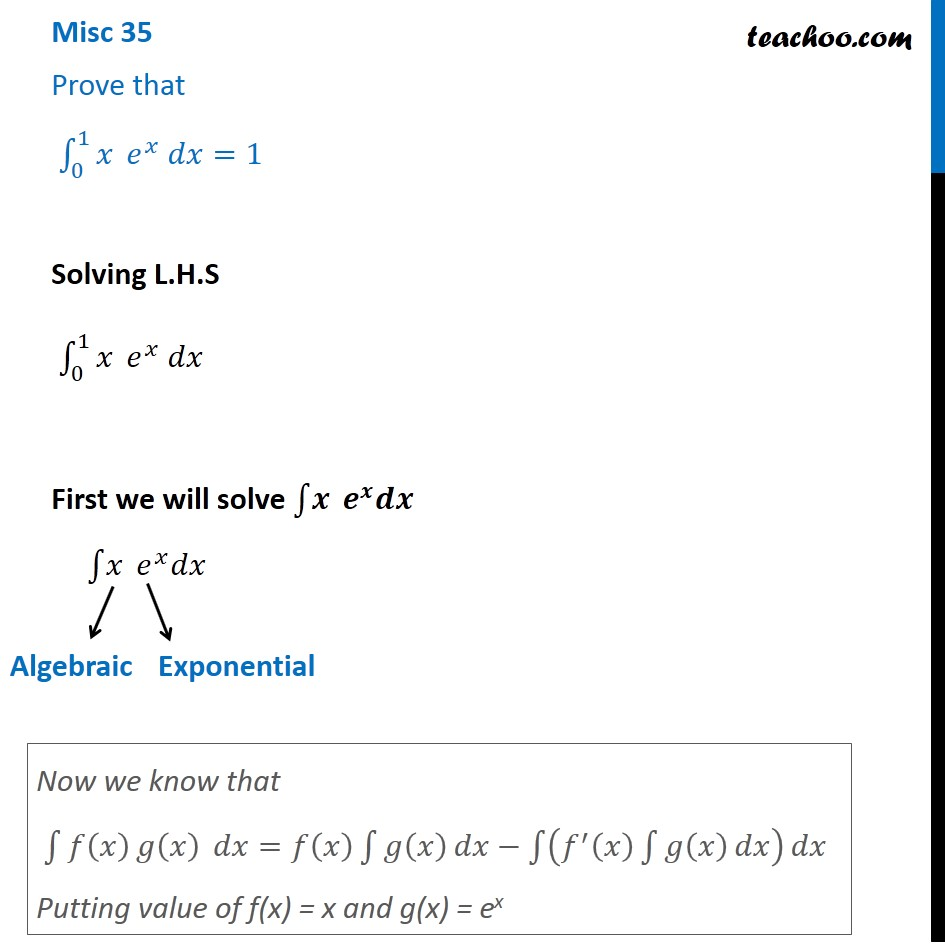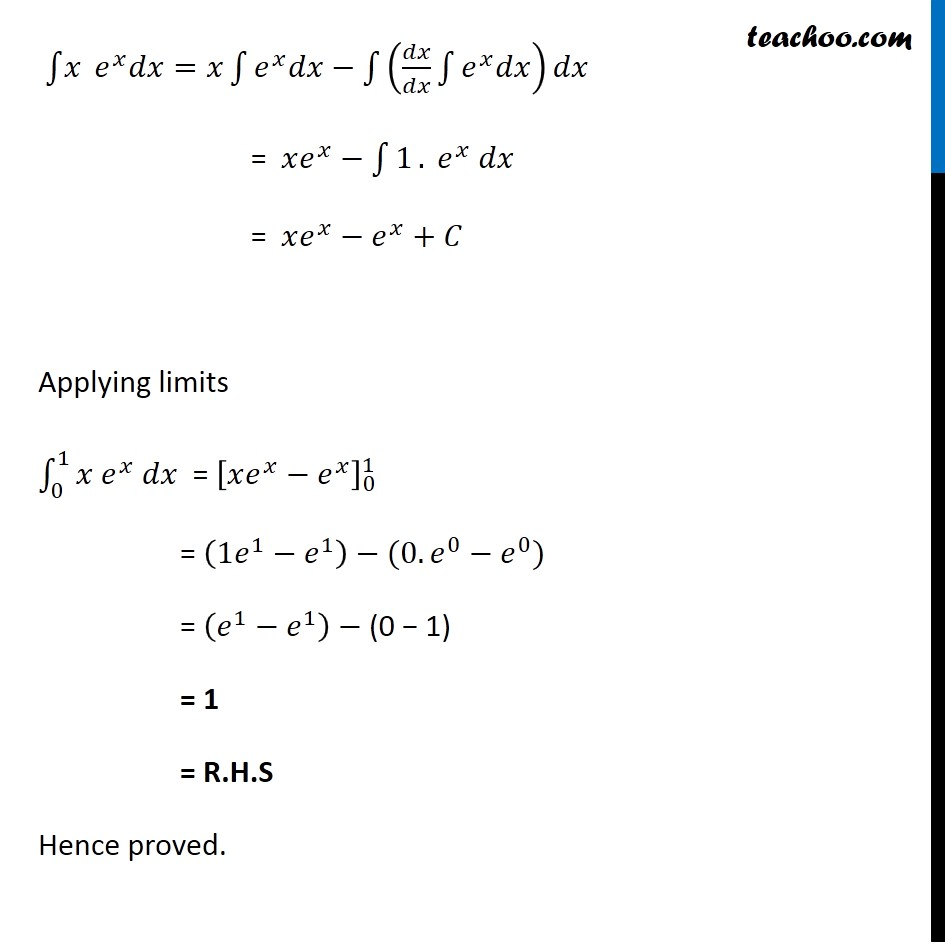Miscellaneous

Chapter 7 Class 12 Integrals
Serial order wiseLearn in your speed, with individual attention - Teachoo Maths 1-on-1 Class

### Transcript

Misc 33 Prove that ∫_0^1▒𝑥 𝑒^𝑥 𝑑𝑥=1 Solving L.H.S ∫_0^1▒𝑥 𝑒^𝑥 𝑑𝑥 First we will solve ∫1▒𝒙 𝒆^𝒙 𝒅𝒙 ∫1▒𝑥 𝑒^𝑥 𝑑𝑥 Now we know that ∫1▒〖𝑓(𝑥) 𝑔⁡(𝑥) 〗 𝑑𝑥=𝑓(𝑥) ∫1▒𝑔(𝑥) 𝑑𝑥−∫1▒(𝑓^′ (𝑥) ∫1▒𝑔(𝑥) 𝑑𝑥) 𝑑𝑥 Putting value of f(x) = x and g(x) = ex ∫1▒𝑥 𝑒^𝑥 𝑑𝑥=𝑥∫1▒〖𝑒^𝑥 𝑑𝑥〗−∫1▒(𝑑𝑥/𝑑𝑥 ∫1▒〖𝑒^𝑥 𝑑𝑥〗) 𝑑𝑥 = 𝑥𝑒^𝑥−∫1▒1. 𝑒^𝑥 𝑑𝑥 = 𝑥𝑒^𝑥−𝑒^𝑥+𝐶 Applying limits ∫1_0^1▒〖𝑥 𝑒^𝑥 𝑑𝑥〗 = [𝑥𝑒^𝑥−𝑒^𝑥 ]_0^1 = (1𝑒^1−𝑒^1 )−(0.𝑒^0−𝑒^0) = (𝑒^1−𝑒^1 )− (0 − 1) = 1 = R.H.S Hence proved.